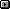FrontpageInjuriesMarathonEventsForums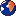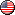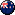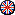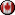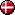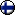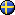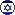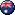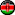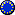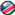# How to calculate the Energy available from foods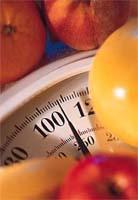To calculate the energy available from a food, multiply the number of grams of carbohydrate, protein, and fat by 4,4, and 9, respectively. Then add the results together.

For example, 1 slice of bread with a tablespoon of peanut butter on it contains 16 grams carbohydrate, 7 grams protein, and 9 grams fat :

16g carbohydrate x 4 kcal/g =      64 kcal
7g protein x 4 kcal/g =      28 kcal
9g fat x 9kcal/g =      81 kcal
Total = 173 kcal

From this information, you can calculate the percentage of kcalories each of the energy nutrients contributes to the total. To determine the percentage of kcalories from fat, for example, divide the 81 fat calories by the total of 173 kcalories:

81 ÷ 173 = 0.468 (rounded to 0.47)

Then multiply by 100 to get the percentage:

0.47 x 100 = 47%

health recommendations that urge people to limit fat intake to 30 percent of kcalories refer to the day’s total energy intake to 30 percent of kcalories refer to the day’s total energy intake, not to individual foods. Still, if the proporation of fat in each food choice throughout a day exceeds 30 percent of kcalories, then the day’s total surely will too. Knowing that this snack provides 47% of its kcalories for fat alerts a person to the need to lower-fat selections at other times of the day.

Also note : 1g alcohol = 7 kcal

The above from : Understanding Nutrition 7th Edition by Eleanor Noss Whitney and Sharon Rady Rolfes

Other articles of interest in the Nutrition section: